Learn all Concepts of Polynomials Class 9 (with VIDEOS). Check - Polynomials Class 9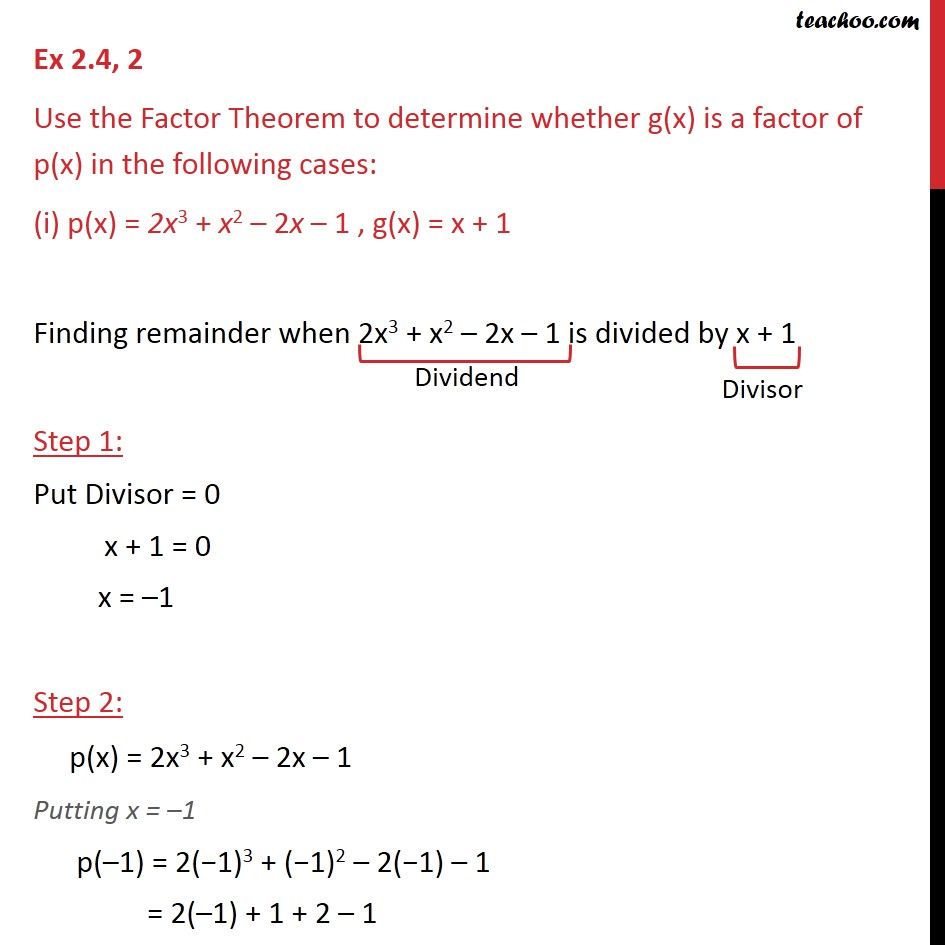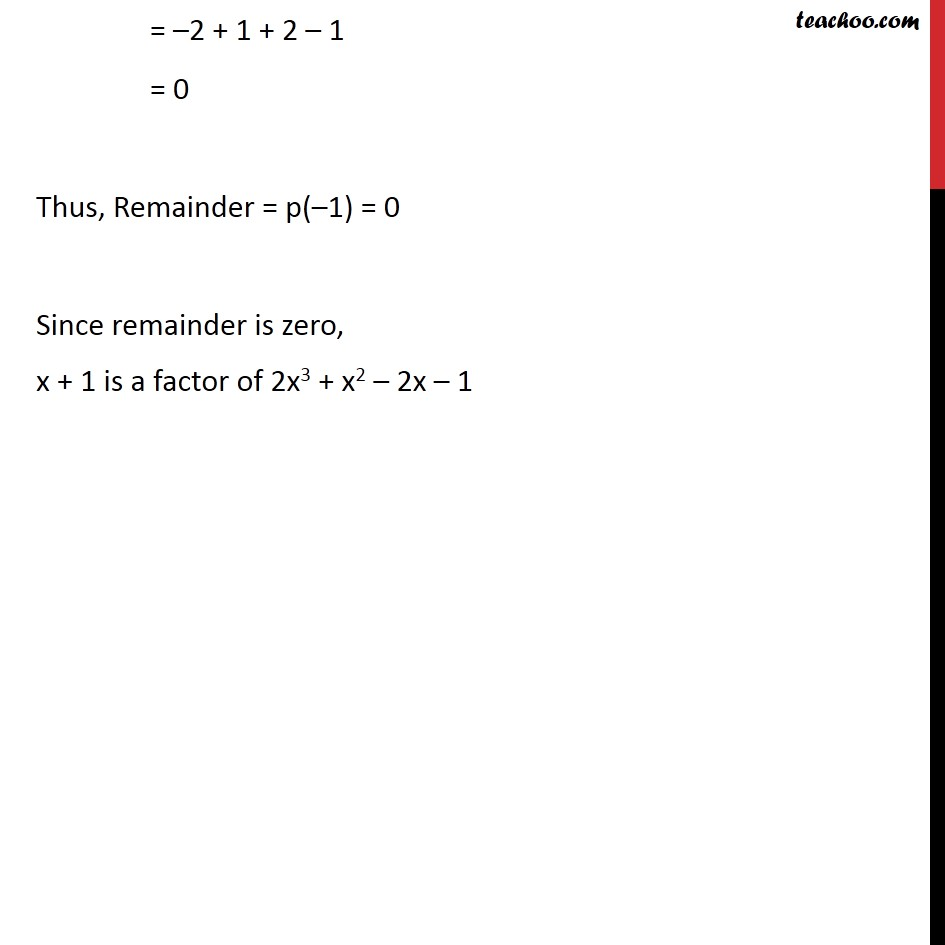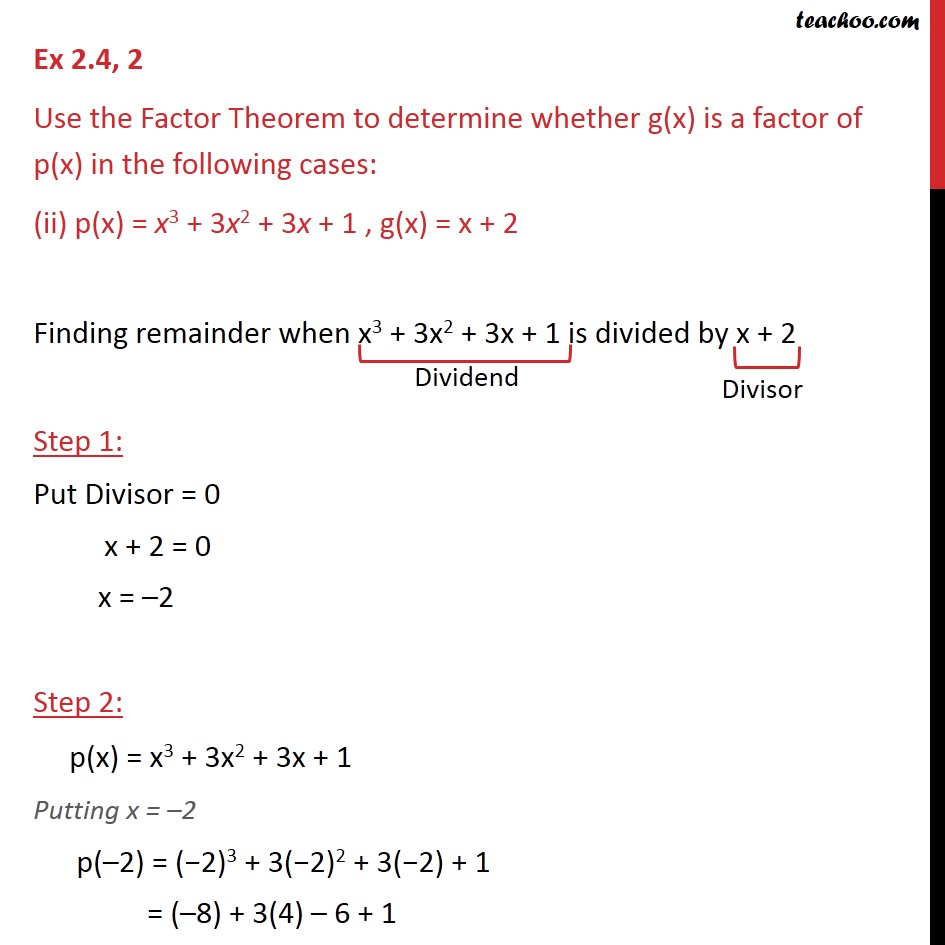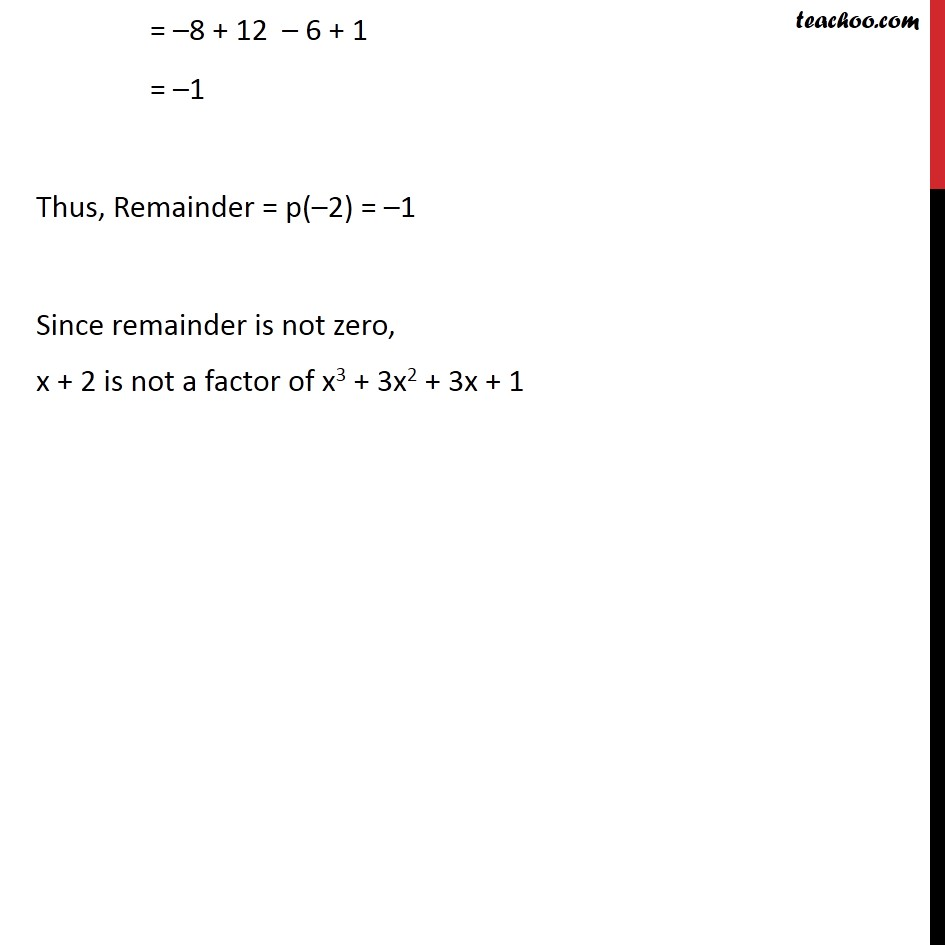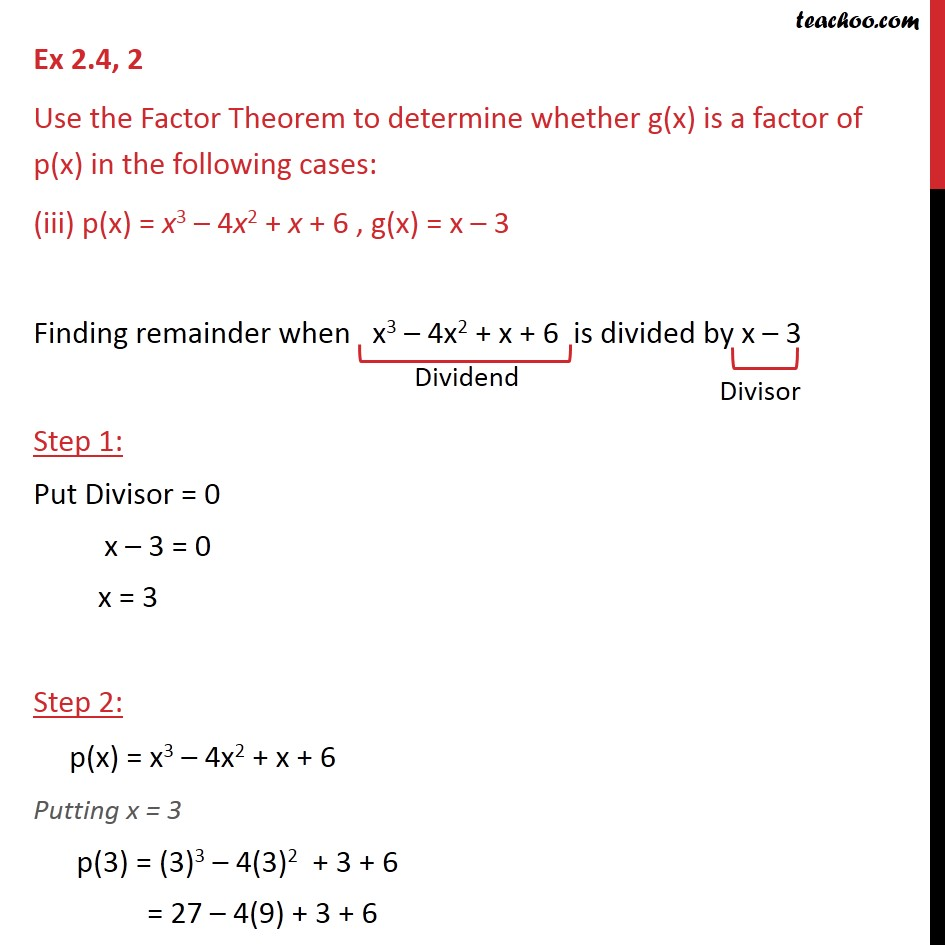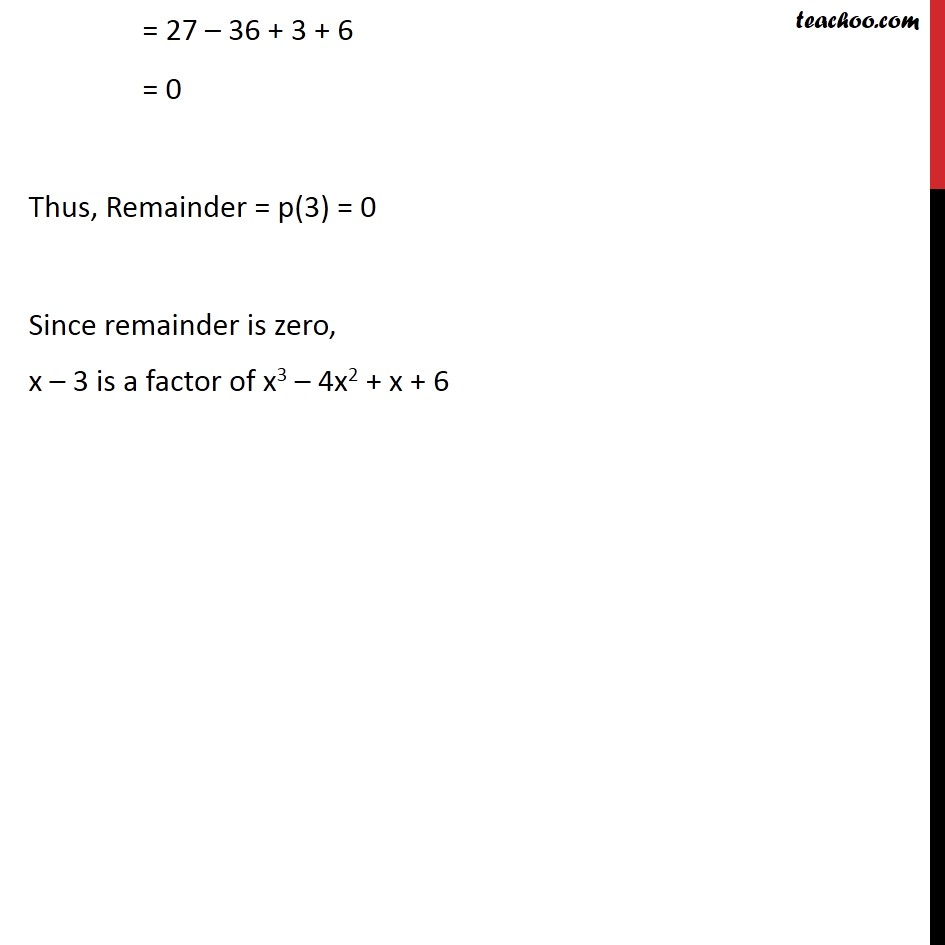1. Chapter 2 Class 9 Polynomials
2. Serial order wise
3. Ex 2.4

Transcript

Ex 2.4, 2 Use the Factor Theorem to determine whether g(x) is a factor of p(x) in the following cases: (i) p(x) = 2x3 + x2 – 2x – 1 , g(x) = x + 1 Finding remainder when 2x3 + x2 – 2x – 1 is divided by x + 1 Step 1: Put Divisor = 0 x + 1 = 0 x = –1 Step 2: p(x) = 2x3 + x2 – 2x – 1 Putting x = –1 p(–1) = 2(−1)3 + (−1)2 – 2(−1) – 1 = 2(–1) + 1 + 2 – 1 = –2 + 1 + 2 – 1 = 0 Thus, Remainder = p(–1) = 0 Since remainder is zero, x + 1 is a factor of 2x3 + x2 – 2x – 1 Ex 2.4, 2 Use the Factor Theorem to determine whether g(x) is a factor of p(x) in the following cases: (ii) p(x) = x3 + 3x2 + 3x + 1 , g(x) = x + 2 Finding remainder when x3 + 3x2 + 3x + 1 is divided by x + 2 Step 1: Put Divisor = 0 x + 2 = 0 x = –2 Step 2: p(x) = x3 + 3x2 + 3x + 1 Putting x = –2 p(–2) = (−2)3 + 3(−2)2 + 3(−2) + 1 = (–8) + 3(4) – 6 + 1 = –8 + 12 – 6 + 1 = –1 Thus, Remainder = p(–2) = –1 Since remainder is not zero, x + 2 is not a factor of x3 + 3x2 + 3x + 1 Ex 2.4, 2 Use the Factor Theorem to determine whether g(x) is a factor of p(x) in the following cases: (iii) p(x) = x3 – 4x2 + x + 6 , g(x) = x – 3 Finding remainder when x3 – 4x2 + x + 6 is divided by x – 3 Step 1: Put Divisor = 0 x – 3 = 0 x = 3 Step 2: p(x) = x3 – 4x2 + x + 6 Putting x = 3 p(3) = (3)3 – 4(3)2 + 3 + 6 = 27 – 4(9) + 3 + 6 = 27 – 36 + 3 + 6 = 0 Thus, Remainder = p(3) = 0 Since remainder is zero, x – 3 is a factor of x3 – 4x2 + x + 6

Ex 2.4

Chapter 2 Class 9 Polynomials
Serial order wise